# We Helped With This MATLAB Programming Homework: Have A Similar One?

SOLVEDCategory Programming MATLAB Undergraduate Solved Matlab Programming Experts

## Short Assignment Requirements

i need all solution with steps for the ten question

## Assignment Description

This is your assignment for this course. All coursework must be submitted in both electronic and paper version

1)      The function move_me is defined like this: function w = move_me(v,a). The first input argument v is a row-vector, while a is a scalar. The function moves every element of v that is equal to a to the end of the vector. For example, the command

>> x = move_me([1 2 3 4],2);

makes x equal to [1 3 4 2]. If a is omitted, the function moves occurrences of zeros.

(5 Marks)

2)      Write a function called halfsum that takes as input an at most two-dimensional array A and computes the sum of the elements of A that are in the lower right triangular part of A, that is, elements in the counter-diagonal (going from the bottom left corner, up and to the right) and elements that are to the right of it. For example, if the input is [1 2; 3 4; 5 6; 7 8], then the function would return 21.

(5 Marks)

3)      Write a function called small_elements that takes as input an array named X that is a matrix or a vector. The function identifies those elements of X that are smaller than the product of their two indexes. For example, if the element X(2,3) is 5, then that element would be identified because 5 is smaller than 2 * 3. The output of the function gives the indexes of such elements found in column-major order. It is a matrix with exactly two columns. The first column contains the row indexes, while the second column contains the corresponding column indexes. For example, the statement indexes = small_elements [1 1; 0 4; 6 5], will make indexes equal to [2 1; 1 2; 3 2]. If no such element exists, the function returns an empty array.

(5 Marks)

4)      Write a function called approximate_e that uses the following formula to compute e, Euler’s number: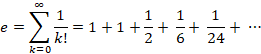Instead of going to infinity, the function stops at the smallest k for which the approximation differs from exp(1) (i.e., the value returned MATLAB’s built-in function) by no more than the positive scalar, delta, which is the only input argument. The first output of the function is the approximate value of e, while the second is k. (Note: if your program or the grader takes a long time, you may have created an infinite loop and need to hit Ctrl-C on your keyboard.) You are not allowed to use the built-in function factorial.

(5 Marks)

5)      Write a function called spiral_diag_sum that takes an odd positive integer n as an input and computes the sum of all the elements in the two diagonals of the n-by-n spiral matrix. For example, starting with the number 1 and moving to the right in a clockwise direction, a 5-by-5 spiral is formed as follows:

 21 22 23 24 25 20 7 8 9 10 19 6 1 2 11 18 5 4 3 12 17 16 15 14 13

The sum of the red elements above is 101. Hint: the problem does not ask for the matrix itself.

(5 Marks)

6)      Write a function called triangle_wave that computes the sum for each of 1001 values of t uniformly spaced from 0 to 4π inclusive. The input argument is a scalar non-negative integer n, and the output argument is a row vector of 1001 such sums—one sum for each value of t. You can test your function by calling it with n == 20 or greater and plotting the result and you will see why the function is called “triangle_wave”.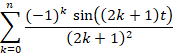(5 Marks)

7)      Write a function max_product that takes v a vector and n, a positive integer, as inputs and computes the largest product of n consecutive elements of v. It returns the product and the index of the element of v that is the first term of the product. If there are multiple such products in v, the function must return the one with the smallest starting index. As an example, the following call will assign 6 to product and 3 to ind since the max 3-term product in the input vector is 2*1*3. If v has fewer than n elements, the function returns 0 and -1, respectively.

>> [product, ind] = max_product([1 2 2 1 3 1],3);

(5 Marks)

8)      Write a function called pendulum that is called like this: T = pendulum(L,a0), where all arguments are scalars and a0 is a positive number less than π. The function calculates the period T of a simple pendulum, which is the time required for a weight attached to a rod of length L and negligible weight to start from rest, swing with no friction under the influence of gravity from an initial angle a0, to – a0 and back to a0 again, as shown in the figure 1. The motion is determined by physics using the following definitions, where units [square brackets] are provided but are not needed: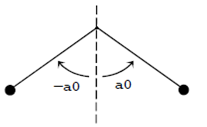Figure 1 Pendulum

g = acceleration due to gravity = 9.8 [m/s2]

t = time [s]

The function starts its calculation with the pendulum angle θ equal to a0 and then calculates a sequence of decreasing pendulum angles, each at a time separated from the one before it by ∆t = 1 × 10-6 s. It continues until the pendulum has passed its lowest point, at which θ = 0. The elapsed time equals T/4.

The calculation at each time step proceeds as follows: The angular acceleration α is set equal to −nsin I⁄L . Then the angular velocity 1 is increased by the product of the angular acceleration and ∆t. That new angular velocity is then used to obtain a new θ by adding the product of the angular velocity and ∆t to the old θ.

Here are two sample runs:

>> format long,

>> T = pendulum(2, pi/2) T =

3.350344000012992

>> T = pendulum(0.22952, pi/4) T =

1.000000000000917

(20 Marks)

9)      Write the function find_zero that is defined like this function x = find_zero(f,x1,x2). The first input argument is special. It is a “function handle”.  A function  handle  is gotten by  typing   @ and   the   name   of   any   function.   For   example,  x = find_zero(@sin,-1,1) will give f the function    handle    for    MATLAB’s    built-in    sin function. Then, inside find_zero, the  statement y = f(-1) would set y = sin(-1). Note that the @ sign is not used inside the function. Only the caller uses it. All other arguments to find_zero are  scalar  numbers, and  x1 is  less  than  x2. The goal of the function is to find an x that lies in the range from x1 to x2 such that after the command,  y = f(x), is executed inside the function find_zero, y is approximately zero as defined by abs(y) < 1e-10. All you know about the function f is that it has one scalar input and one scalar output, and a plot of its values crosses the x-axis exactly once between x1 and x2, as, for example, in the figure 3. It is the responsibility of the caller to call the function with arguments that obey these rules.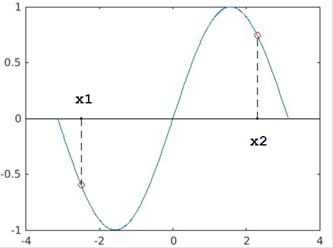Figure 3 Output plot for x1 and x2 (Hint: Remember to label your axis’s)

Here are two sample runs:

>> find_zero(@sin,-2.5,2.3) % as shown in the figure

ans =

-6.4000e-11

>> format long

>> find_zero(@cos,-2,1.3)

ans =

-1.570796326871000

(20 Marks)

10)  Write a function that is called like this [E,N] = cyclotron(V). All arguments are scalars. The input argument is the voltage applied to a cyclotron (figure 2), which is a device that accelerates subatomic particles—in this case, positively charged isotopes of hydrogen, called “deuterons”—which spiral outward in a clockwise direction. The cyclotron rapidly alternates the sign of the voltage difference V in units of volts between two “D”-shaped vacuum chambers (blue outlines), which are placed within a strong uniform magnetic field (not shown but perpendicular to the page). The deuteron is accelerated only as it is leaving one “D” and entering the other. While the deuteron is inside a given “D”, it moves at a constant speed, and the magnetic field causes it to move on a semicircle. Each deuteron moves as follows (check the numbers in the figure 2):

i)       It originates from a source (red dot) located at a distance s0 to the left of the centre of the cyclotron, is accelerated vertically into the upper “D” and then moves on a semi-circle of radius r1 .

ii)     It leaves the upper “D” and is accelerated vertically downward into the lower “D” where moves with a larger radius r2.

iii)   It leaves the lower “D” and is accelerated vertically into the upper “D”, etc, moving with ever increasing radii rn until (N) it is accelerated for the final time as it leaves the upper “D” and enters the lower “D”, follows a semicircle of radius rN, and emerges from the cyclotron at the left.

iv)   The formulas for the radii are as follows:

(1)  r1 =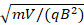,

(2)   m = deuteron mass = 3.344×10-27 kg,

(3)   q = deuteron charge = 1.603×10-19 coulomb, and

v)      B = magnetic field strength = 1.600 tesla.

vi)   For ≥ 2 ,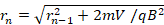.

vii) These expressions give the radii in units of meters, and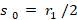.

The deuteron escapes through a window at the left that is placed so the particle cannot leave until it is more than 0.500 m to the left of the centre of the cyclotron. The gap between the “D”s is exaggerated in the figure, has no effect, and can be assumed to be of zero width. The function returns energy E of the deuteron when it escapes in units of million electron volts (MeV), which equals VN×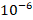, and the number N of times the deuteron enters the “D”s. HINT: Notice that the centres of the semicircles ≠ the centre of the cyclotron.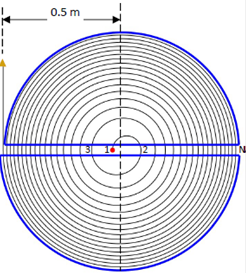Figure 2 Cyclotron. A charged particle emerges from a source near the centre, spirals out while accelerating to high speed, and emerges at the left.

Here is a sample run:

>> [E, N] = cyclotron(4.8e5) % depicted in figure

E =

16.32

N =

34

(25 Marks)

Is it free to get my assignment evaluated?

Yes. No hidden fees. You pay for the solution only, and all the explanations about how to run it are included in the price. It takes up to 24 hours to get a quote from an expert. In some cases, we can help you faster if an expert is available, but you should always order in advance to avoid the risks. You can place a new order here.

How much does it cost?

The cost depends on many factors: how far away the deadline is, how hard/big the task is, if it is code only or a report, etc. We try to give rough estimates here, but it is just for orientation (in USD):

 Regular homework \$20 - \$150 Advanced homework \$100 - \$300 Group project or a report \$200 - \$500 Mid-term or final project \$200 - \$800 Live exam help \$100 - \$300 Full thesis \$1000 - \$3000

How do I pay?

Credit card or PayPal. You don't need to create/have a Payal account in order to pay by a credit card. Paypal offers you "buyer's protection" in case of any issues.

Why do I need to pay in advance?

We have no way to request money after we send you the solution. PayPal works as a middleman, which protects you in case of any disputes, so you should feel safe paying using PayPal.

Do you do essays?

No, unless it is a data analysis essay or report. This is because essays are very personal and it is easy to see when they are written by another person. This is not the case with math and programming.

Why there are no discounts?

It is because we don't want to lie - in such services no discount can be set in advance because we set the price knowing that there is a discount. For example, if we wanted to ask for \$100, we could tell that the price is \$200 and because you are special, we can do a 50% discount. It is the way all scam websites operate. We set honest prices instead, so there is no need for fake discounts.

Do you do live tutoring?

No, it is simply not how we operate. How often do you meet a great programmer who is also a great speaker? Rarely. It is why we encourage our experts to write down explanations instead of having a live call. It is often enough to get you started - analyzing and running the solutions is a big part of learning.

What happens if I am not satisfied with the solution?

Another expert will review the task, and if your claim is reasonable - we refund the payment and often block the freelancer from our platform. Because we are so harsh with our experts - the ones working with us are very trustworthy to deliver high-quality assignment solutions on time.

Customer Feedback

"Thanks for explanations after the assignment was already completed... Emily is such a nice tutor! "

Order #13073